# Gaussian Integrals

(edited by Roberto Bigoni)

The integrals of the form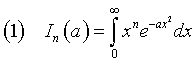which are frequently encountered in the probability theory, considered as functions of a, have the following recursive property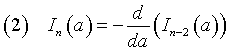that is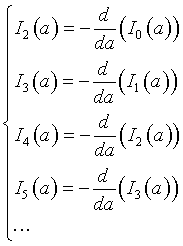Therefore, if we know Io(a) and I1(a), we can calculate any other In(a).

• The calculation of I1(a) is quite easy.
In this case the integrand function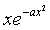is the derivative with respect to x of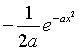so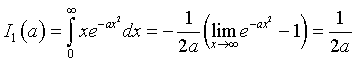• The value of Io(a), in which the integrand function is the exponential function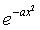can be obtained in the following way:

1. we consider twice the integral of this function on the whole ℜ, using two variables x and y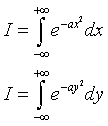therefore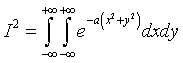2. in the plane Oxy we substitute the polar coordinates (ρ,θ) to the Cartesian coordinates (x,y):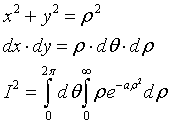3. the first integral of the product has value , the second is I1, therefore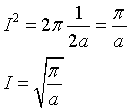4. Due to the symmetry of the Gaussian function, the value of I0 is half of this value, therefore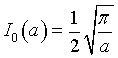Finally we can obtain from (2)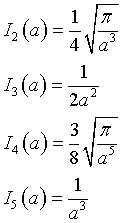and so on.

In general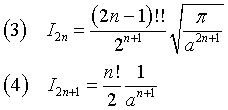that is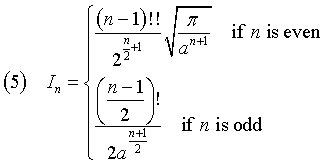last revision: May 2018